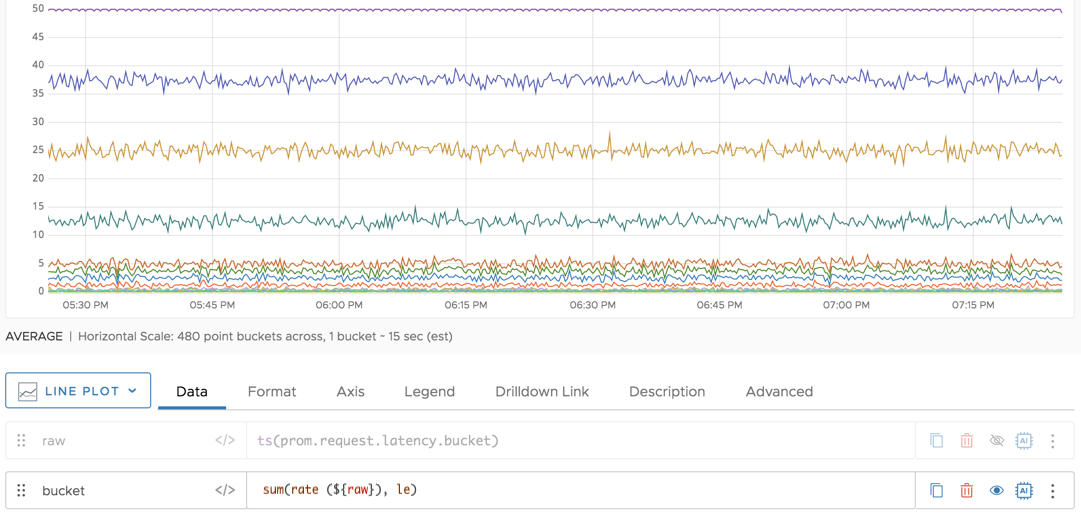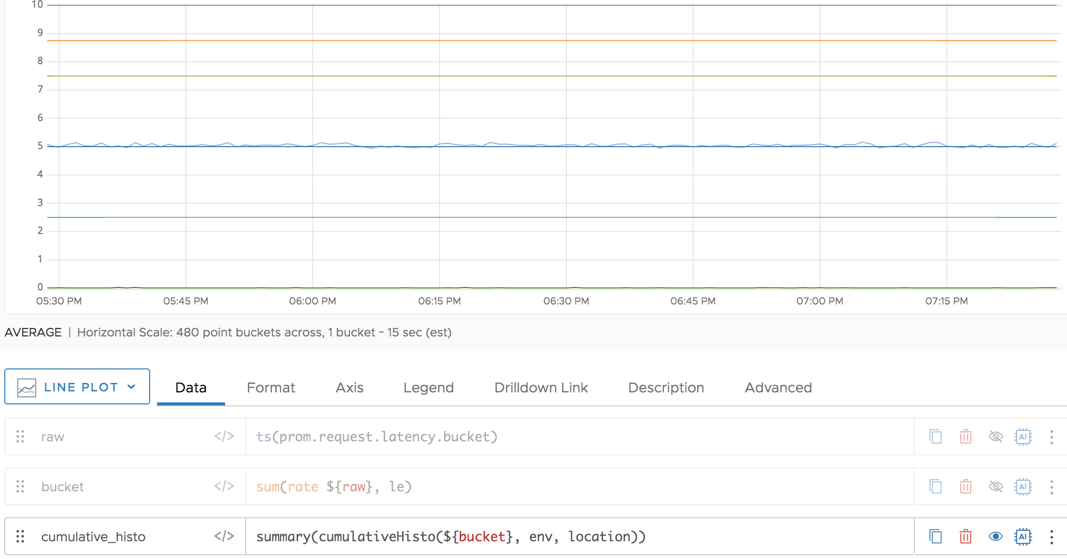Reference to the cumulativeHisto() function. Convert Prometheus cumulative histograms to Wavefront ordinary histograms.

## Summary

``````cumulativeHisto([<timeWindow>,] [<bucketName>,] <tsExpression>
[,metrics|sources|sourceTags|pointTags|<pointTagKey>] )
``````

Converts a cumulative histogram coming from Prometheus, Telegraf, or other source to an ordinary histogram in Wavefront histogram format. Users can then manipulate the histogram with Wavefront histogram query functions.

## Parameters

ParameterDescription
timeWindow Amount of time in the moving time window. You can specify a time measurement based on the clock or calendar (1s, 1m, 1h, 1d, 1w), the window length (1vw) of the chart, or the bucket size (1bw) of the chart. Defaults to 1m.
bucketName Optional string that describes the bucket. Default is le, that is, less than or equal. If your source histogram uses a different tag key to specify the buckets, specify that tag key here.
tsExpression Cumulative histogram that we'll convert to an ordinary histogram.
metrics|sources|sourceTags|pointTags|<pointTagKey> Optional 'group by' parameter for organizing the time series into subgroups and then returning each histogram subgroup. Use one or more parameters to group by metric names, source names, source tag names, point tag names, values for a particular point tag key, or any combination of these items. Specify point tag keys by name.

## Description

This function is useful if you want to analyze data that are already in a cumulative histogram format.

This function works only with data that include a parameter such as `le` for defining which part of the cumulative histogram you want to display. Data that are imported from Prometheus always include such a parameter.

When a chart displays the result of this function, it shows the median by default. You can use `percentile()` to change that and, for example, show the 90% percentile.

### Ordinary and Cumulative Histograms

Wavefront histogram distributions are ordinary histograms while some other tools, such as Prometheus and Telegraf, use cumulative histograms.(image credit: Wikipedia)

If your data source emits cumulative histograms, you can use this function to visualize your histogram data in Wavefront.

### How to Map Prometheus Queries to Wavefront Queries

When you use Prometheus, you run queries like this:

``````histogram_quantile(0.90, sum(rate(req_latency_bucket[5m])) by (le))
``````

This query displays the 90th quantile of a cumulative histogram that corresponds to the `req_latency_bucket` metric. The `le` parameter means `less than or equal.

The corresponding Wavefront query looks like this:

``````percentile(90, cumulativeHisto(sum(rate(ts(req_latency_bucket)), le)))
``````

Here, we are creating a T-digest and adding sampling points based on the range and the count of the bucket.

### Grouping

Similar to aggregation functions for metrics, `cumulativeHisto()` returns a single distribution per specified time window. To get separate distributions for groups that share common characteristics, you can include a ‘group by’ parameter, as for many ts() queries. For example, use `cumulativeHisto(1m, <expression>, sources)` to group by sources.

The function returns a separate series of results for each group.

### Interpolation

The `cumulativeHisto()` function itself doesn’t perform interpolation because that doesn’t make sense for a histogram. But when you apply `percentile()`, we do perform interpolation.

## Example

The following example starts with a cumulative histogram in Prometheus format. We can show only histogram values that are less than or equal to 60 using the `le` tag. You can see the `le` tag in the legend.We can then manipulate the cumulative histogram. First, we use `sum(rate())` to return the per-second rate of each time series.Then we use the `cumulativeHisto()` function to return the cumulative histogram for the data.